# making some columns more important

Member, KB Contributor Posts: 128Guru
edited May 12 in Help
سلام. وقت بخیر
چگونه میتوان بعضی از ستون های اکسل را در بکارگیری مدل موثرتر و کارآمد تر بیان کرد؟
--------------------------------------------------------------------------------------------------------------------------------------------------------------------
Hello
how we can make some columns more important and effective?
Tagged:

## Best Answer

• Posts: 394Unicorn
Solution Accepted
Hello @mbs:

You are connecting these the wrong way.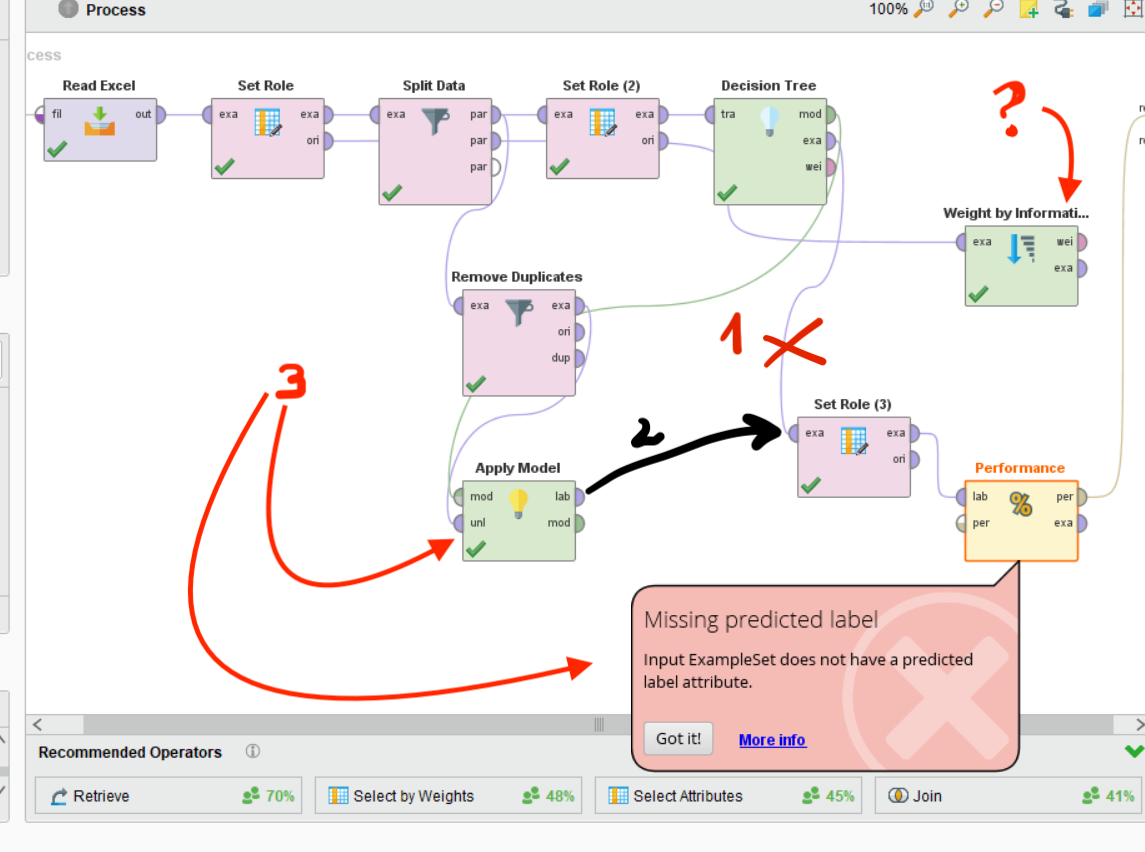The line marked with an X and a 1, going between the exa output in the Decision Tree operator and the exa input in the Set Role operator shouldn't be there. Instead, replace it with the black line marked with a 2, because the predicted label is added by the Apply Model operator when you apply a model through the mod input and a set of not labeled data in the unl input.

First, let's fix this and then we can continue with weightlifting weight handling. I am setting up an example for you.

## Answers

• Member Posts: 661Unicorn
Hello @mbs

Can you please explain more about your requirement?
• Member, KB Contributor Posts: 128Guru
edited May 12
@varunm1

I mean that I want make some columns more effective on the algorithm.
some columns are more important Features so  i need to make them more effective
thank you• Member, KB Contributor Posts: 128Guru
edited May 12
and I did this but it doesnt work
it has label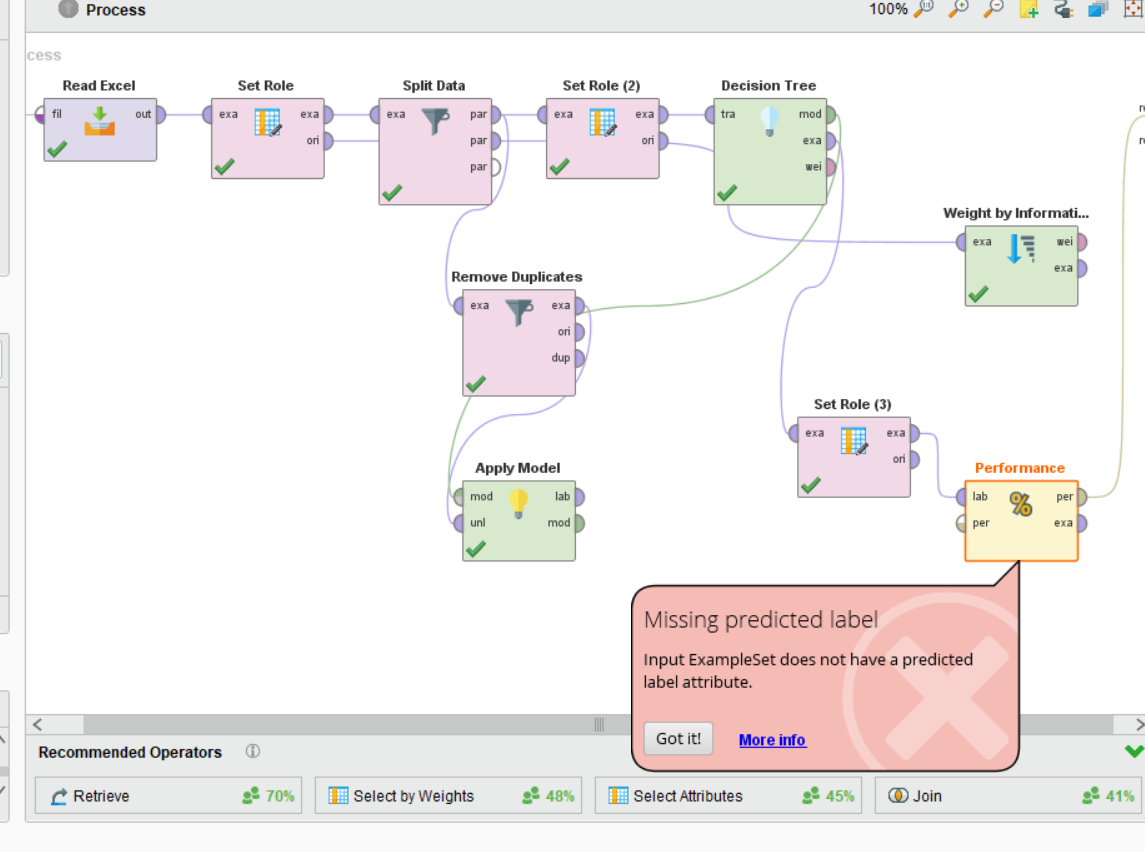• Member, KB Contributor Posts: 128Guru
edited May 12
;rfuentealba
thank you for your help I will try it and then for weight if I have any problem I will ask
also is weighting good way for making some columns more effective?
mbs
• Moderator, RapidMiner Certified Analyst, Member, University Professor Posts: 394Unicorn
Hello @mbs,

Here is your example on how to Select by Weights. There are some more things you should know, but first: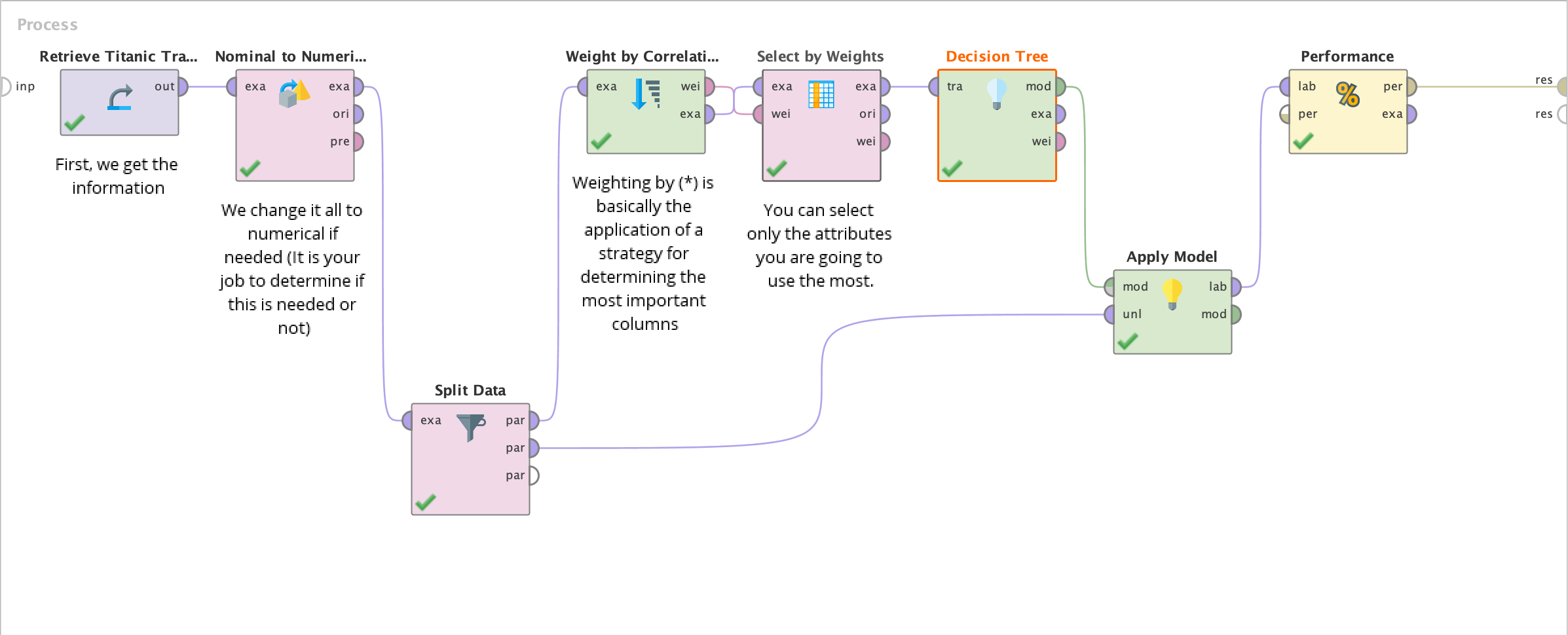• I convert everything to Numerical because weighting can't be applied to categories.
• Splitting the data stratifying the examples.
• Applying the weighting by correlation method to the stratified examples. You can select any kind of weighting at this point.
• Selecting the most important weights to train our Decision Tree.
• The rest is standard procedure.
You may also want to use Decision Tree (Weight Based) or DBSCAN (Weight Based), as not all ML algorithms support weight-based operations.

Now, this process takes only the most important weighted columns, and discard the others. Here is the XML, in case you wish to experiment:

```<?xml version="1.0" encoding="UTF-8"?><process version="9.2.001">
<context>
<input/>
<output/>
<macros/>
</context>
<operator activated="true" class="process" compatibility="9.2.001" expanded="true" name="Process">
<parameter key="logverbosity" value="init"/>
<parameter key="random_seed" value="2001"/>
<parameter key="send_mail" value="never"/>
<parameter key="notification_email" value=""/>
<parameter key="process_duration_for_mail" value="30"/>
<parameter key="encoding" value="SYSTEM"/>
<process expanded="true">
<operator activated="true" class="retrieve" compatibility="9.2.001" expanded="true" height="68" name="Retrieve Titanic Training" width="90" x="45" y="34">
<parameter key="repository_entry" value="//Samples/data/Titanic Training"/>
<description align="center" color="transparent" colored="false" width="126">First, we get the information</description>
</operator>
<operator activated="true" class="nominal_to_numerical" compatibility="9.2.001" expanded="true" height="103" name="Nominal to Numerical" width="90" x="179" y="34">
<parameter key="return_preprocessing_model" value="false"/>
<parameter key="create_view" value="true"/>
<parameter key="attribute_filter_type" value="subset"/>
<parameter key="attribute" value=""/>
<parameter key="attributes" value="Passenger Class|Sex"/>
<parameter key="use_except_expression" value="false"/>
<parameter key="value_type" value="nominal"/>
<parameter key="use_value_type_exception" value="false"/>
<parameter key="except_value_type" value="file_path"/>
<parameter key="block_type" value="single_value"/>
<parameter key="use_block_type_exception" value="false"/>
<parameter key="except_block_type" value="single_value"/>
<parameter key="invert_selection" value="false"/>
<parameter key="include_special_attributes" value="false"/>
<parameter key="coding_type" value="unique integers"/>
<parameter key="use_comparison_groups" value="false"/>
<list key="comparison_groups"/>
<parameter key="unexpected_value_handling" value="all 0 and warning"/>
<parameter key="use_underscore_in_name" value="false"/>
<description align="center" color="transparent" colored="false" width="126">We change it all to numerical if needed (It is your job to determine if this is needed or not)</description>
</operator>
<operator activated="true" class="split_data" compatibility="9.2.001" expanded="true" height="103" name="Split Data" width="90" x="313" y="289">
<enumeration key="partitions">
<parameter key="ratio" value="0.8"/>
<parameter key="ratio" value="0.2"/>
</enumeration>
<parameter key="sampling_type" value="stratified sampling"/>
<parameter key="use_local_random_seed" value="false"/>
<parameter key="local_random_seed" value="1992"/>
</operator>
<operator activated="true" class="weight_by_correlation" compatibility="9.2.001" expanded="true" height="82" name="Weight by Correlation" width="90" x="447" y="34">
<parameter key="normalize_weights" value="true"/>
<parameter key="sort_weights" value="true"/>
<parameter key="sort_direction" value="ascending"/>
<parameter key="squared_correlation" value="true"/>
<description align="center" color="transparent" colored="false" width="126">Weighting by (*) is basically the application of a strategy for determining the most important columns</description>
</operator>
<operator activated="true" class="select_by_weights" compatibility="9.2.001" expanded="true" height="103" name="Select by Weights" width="90" x="581" y="34">
<parameter key="weight_relation" value="top p%"/>
<parameter key="weight" value="1.0"/>
<parameter key="k" value="5"/>
<parameter key="p" value="0.5"/>
<parameter key="deselect_unknown" value="true"/>
<parameter key="use_absolute_weights" value="true"/>
<description align="center" color="transparent" colored="false" width="126">You can select only the attributes you are going to use the most.</description>
</operator>
<operator activated="true" class="concurrency:parallel_decision_tree" compatibility="9.2.001" expanded="true" height="103" name="Decision Tree" width="90" x="715" y="34">
<parameter key="criterion" value="accuracy"/>
<parameter key="maximal_depth" value="5"/>
<parameter key="apply_pruning" value="true"/>
<parameter key="confidence" value="0.2"/>
<parameter key="apply_prepruning" value="true"/>
<parameter key="minimal_gain" value="0.01"/>
<parameter key="minimal_leaf_size" value="2"/>
<parameter key="minimal_size_for_split" value="4"/>
<parameter key="number_of_prepruning_alternatives" value="3"/>
</operator>
<operator activated="true" class="apply_model" compatibility="9.2.001" expanded="true" height="82" name="Apply Model" width="90" x="849" y="187">
<list key="application_parameters"/>
<parameter key="create_view" value="false"/>
</operator>
<operator activated="true" class="performance_classification" compatibility="9.2.001" expanded="true" height="82" name="Performance" width="90" x="983" y="34">
<parameter key="main_criterion" value="first"/>
<parameter key="accuracy" value="true"/>
<parameter key="classification_error" value="false"/>
<parameter key="kappa" value="false"/>
<parameter key="weighted_mean_recall" value="false"/>
<parameter key="weighted_mean_precision" value="false"/>
<parameter key="spearman_rho" value="false"/>
<parameter key="kendall_tau" value="false"/>
<parameter key="absolute_error" value="false"/>
<parameter key="relative_error" value="false"/>
<parameter key="relative_error_lenient" value="false"/>
<parameter key="relative_error_strict" value="false"/>
<parameter key="normalized_absolute_error" value="false"/>
<parameter key="root_mean_squared_error" value="false"/>
<parameter key="root_relative_squared_error" value="false"/>
<parameter key="squared_error" value="false"/>
<parameter key="correlation" value="false"/>
<parameter key="squared_correlation" value="false"/>
<parameter key="cross-entropy" value="false"/>
<parameter key="margin" value="false"/>
<parameter key="soft_margin_loss" value="false"/>
<parameter key="logistic_loss" value="false"/>
<parameter key="skip_undefined_labels" value="true"/>
<parameter key="use_example_weights" value="true"/>
<list key="class_weights"/>
</operator>
<connect from_op="Retrieve Titanic Training" from_port="output" to_op="Nominal to Numerical" to_port="example set input"/>
<connect from_op="Nominal to Numerical" from_port="example set output" to_op="Split Data" to_port="example set"/>
<connect from_op="Split Data" from_port="partition 1" to_op="Weight by Correlation" to_port="example set"/>
<connect from_op="Split Data" from_port="partition 2" to_op="Apply Model" to_port="unlabelled data"/>
<connect from_op="Weight by Correlation" from_port="weights" to_op="Select by Weights" to_port="weights"/>
<connect from_op="Weight by Correlation" from_port="example set" to_op="Select by Weights" to_port="example set input"/>
<connect from_op="Select by Weights" from_port="example set output" to_op="Decision Tree" to_port="training set"/>
<connect from_op="Decision Tree" from_port="model" to_op="Apply Model" to_port="model"/>
<connect from_op="Apply Model" from_port="labelled data" to_op="Performance" to_port="labelled data"/>
<connect from_op="Performance" from_port="performance" to_port="result 1"/>
<portSpacing port="source_input 1" spacing="0"/>
<portSpacing port="sink_result 1" spacing="0"/>
<portSpacing port="sink_result 2" spacing="0"/>
</process>
</operator>
</process>```

Hope this helps.

• Moderator, RapidMiner Certified Analyst, Member, University Professor Posts: 394Unicorn
@mbs,

Yes, it's very recommended to use weighting for this. Most of the time, it's even more recommended than upsampling or downsampling.

All the best,

Rodrigo.
• Member, KB Contributor Posts: 128Guru
@rfuentealba
thank you very much for your help• Member, KB Contributor Posts: 128Guru
@rfuentealba
With your example I change the data with my dataset but I have to add some more operator in order to process work with my data. please look at these screen shots and also I can not understand the resultAny way thank you for your help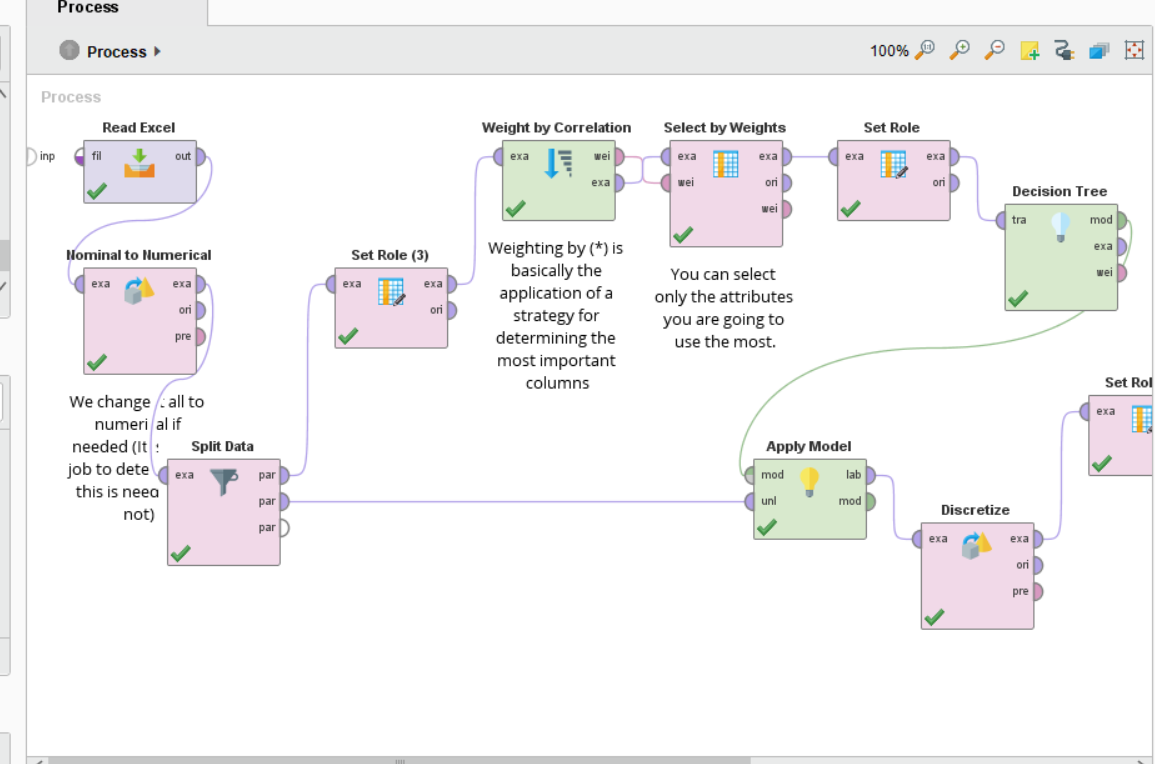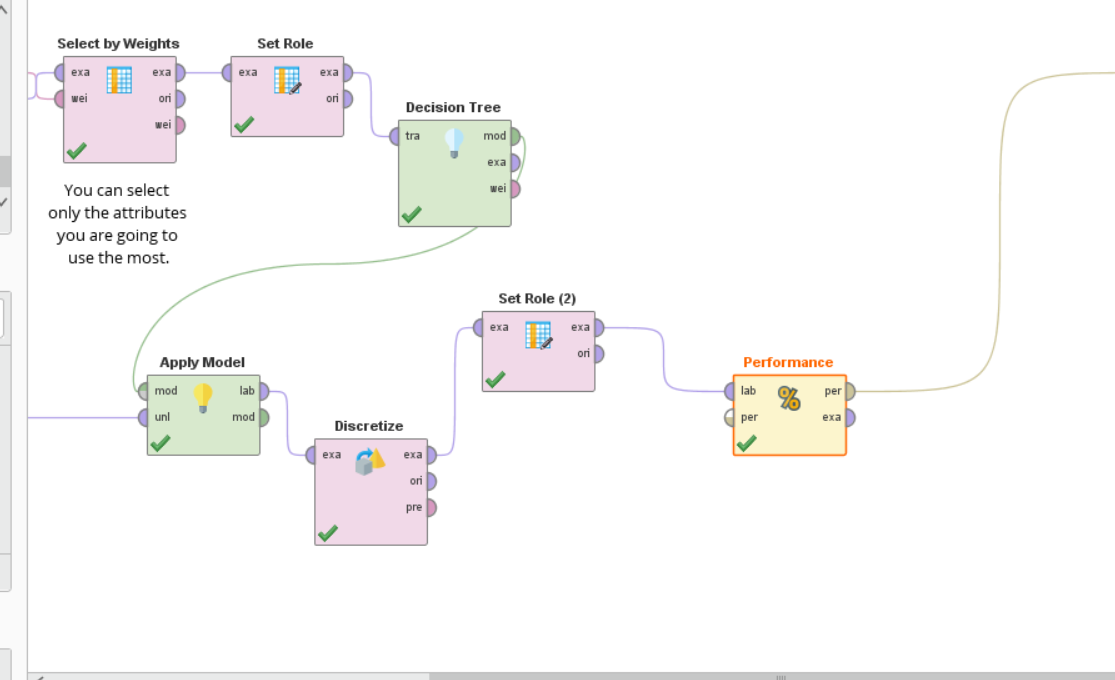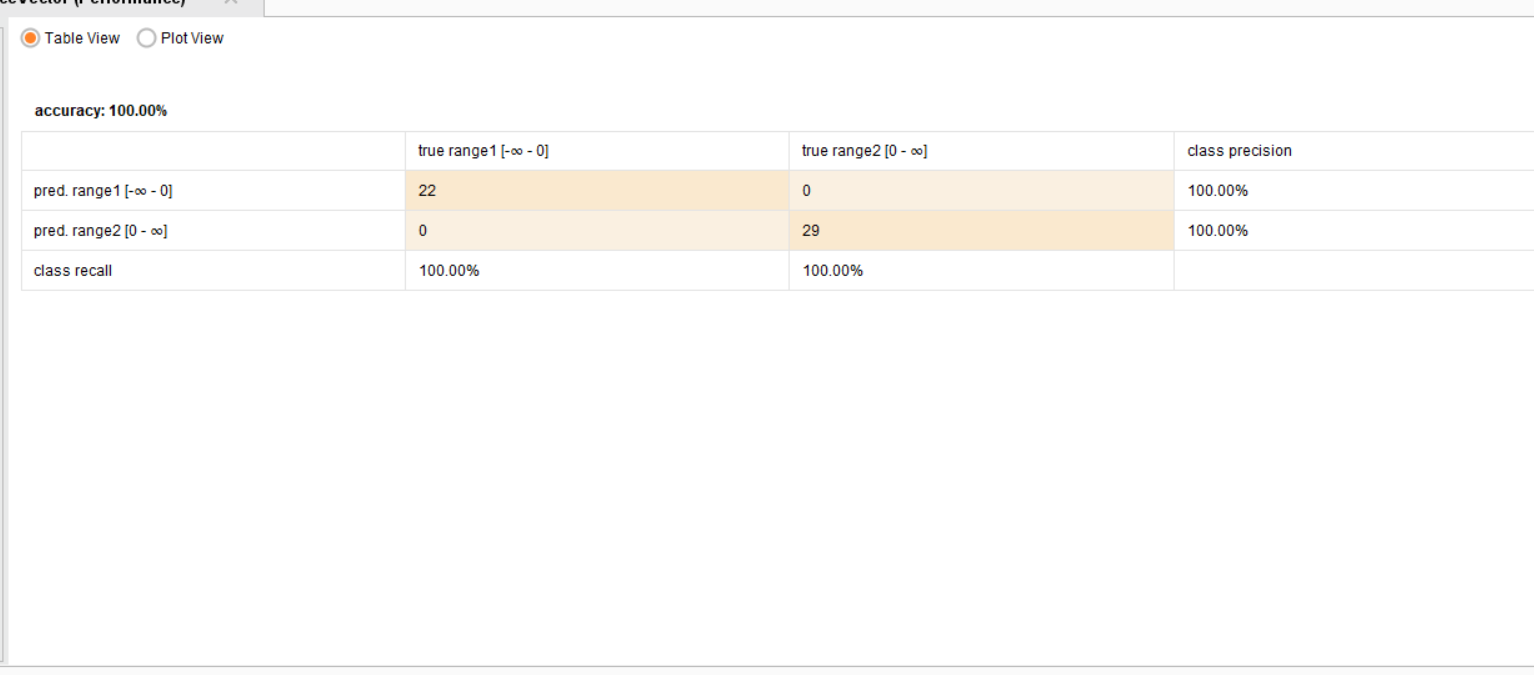• Member, KB Contributor Posts: 128Guru
@rfuentealba
the points that you mentioned in the screen shot works but still i use weighting by information gain because the correlation operator doesnt work with my data and also I changed tree to the ruleinduction and the result is 98.86thank you
• Moderator, RapidMiner Certified Analyst, Member, University Professor Posts: 394Unicorn
Hello @mbs,

To be clear: my example was a quick one to show the specific ordering of the elements. If you want to do weighting by awesomeness, go ahead, hahaha.

The result is a confusion matrix or something, where you need to see:
• How many predicted positives are in the true positives list?
• How many predicted negatives are in the true negatives list?
• The class precision = how precisely can you select the sampled true positives (or sampled true negatives)
• The class recall = how precisely can you select all the true positives (or true negatives)
In the case you showed, 100% is a perfect score. The problem would be if with that 100%-scored algorithm you can score the 100% of the new phenomena, because if it doesn't, you are causing overfitting to your model.All the best,

Rodrigo.
• Member, KB Contributor Posts: 128Guru
hello
@rfuentealba
with your example and my data i can not understand the result and it is not clear. but with my example every thing is clear
Sign In or Register to comment.